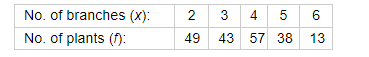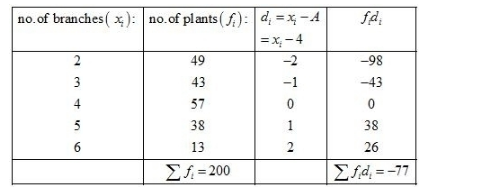# The following table given the number of branches and number of plants in the garden of a school.

Question:

The following table given the number of branches and number of plants in the garden of a school.Solution:

Let the assume mean be $A=4$.We know that mean, $\bar{X}=A+\frac{1}{N} \sum_{i=1}^{n} f_{i} d_{i}$

Now, we have $N=\sum f_{i}=200, \sum f_{i} d_{i}=-77$ and $A=4$

Putting the values in the above formula, we get

$\bar{X}=A+\frac{1}{N} \sum_{i=1}^{n} f_{i} d_{i}$

$=4+\frac{1}{200} \times(-77)$

$=4-0.385$

$=3.615$

$\approx 3.62$ ( approximate)

Hence, the mean number of branches per plant is approximately 3.62.